# 使用机器学习来预测股票价格

+关注继续查看#### 工作流程

1.获取股票价格数据；

2.使用小波变换去噪数据；

3.使用Stacked Autoencoders提取特征；

4.使用特征训练LSTM

5.测试预测准确性的模型；

#### 1.数据采集

pandas_datareader是借助雅虎财经的API，它可以很容易获得股票价格数据，只需使用以下命令即可完成：

stock_data = pdr.get_data_yahoo(self.ticker, self.start, self.end)

#### 小波变换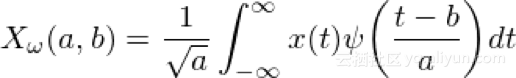1.使用小波变换变换数据；

2.消除超完全准偏差的系数（在所有系数中）；

3.变换新系数以得去噪数据；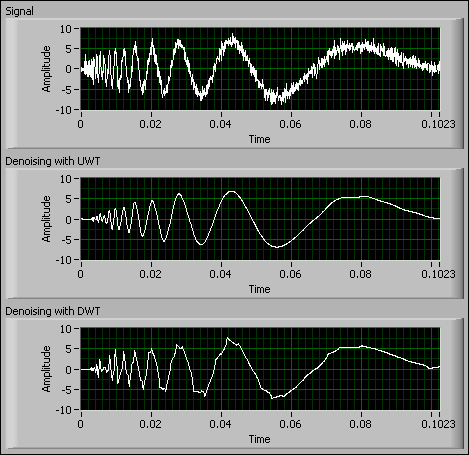x = np.array(self.stock_data.iloc[i: i + 11, j])
(ca, cd) = pywt.dwt(x, "haar")
cat = pywt.threshold(ca, np.std(ca), mode="soft")
cdt = pywt.threshold(cd, np.std(cd), mode="soft")
tx = pywt.idwt(cat, cdt, "haar")

#### 堆叠式自动编码器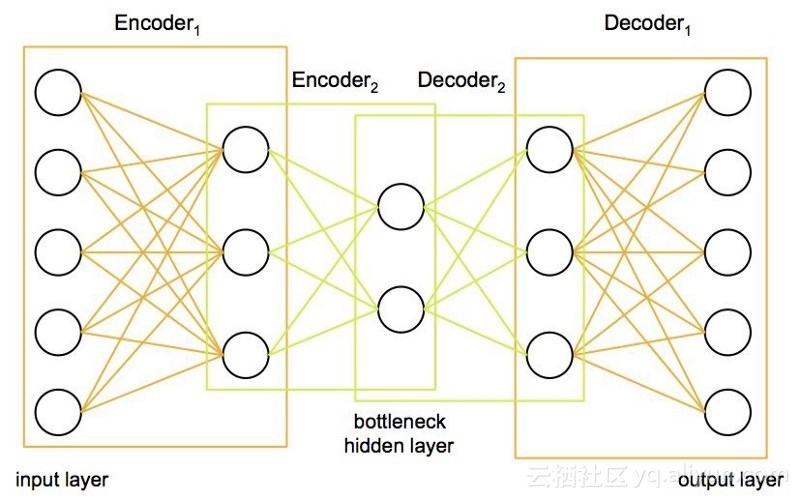class AutoEncoder:
def __init__(self, encoding_dim):
self.encoding_dim = encoding_dim
def build_train_model(self, input_shape, encoded1_shape, encoded2_shape, decoded1_shape, decoded2_shape):
input_data = Input(shape=(1, input_shape))
encoded1 = Dense(encoded1_shape, activation="relu", activity_regularizer=regularizers.l2(0))(input_data)
encoded2 = Dense(encoded2_shape, activation="relu", activity_regularizer=regularizers.l2(0))(encoded1)
encoded3 = Dense(self.encoding_dim, activation="relu", activity_regularizer=regularizers.l2(0))(encoded2)
decoded1 = Dense(decoded1_shape, activation="relu", activity_regularizer=regularizers.l2(0))(encoded3)
decoded2 = Dense(decoded2_shape, activation="relu", activity_regularizer=regularizers.l2(0))(decoded1)
decoded = Dense(input_shape, activation="sigmoid", activity_regularizer=regularizers.l2(0))(decoded2)
autoencoder = Model(inputs=input_data, outputs=decoded)
encoder = Model(input_data, encoded3)
# Now train the model using data we already preprocessed
ntrain = np.array(train)
train_data = np.reshape(ntrain, (len(ntrain), 1, input_shape))
# print(train_data)
# autoencoder.summary()
autoencoder.fit(train_data, train_data, epochs=1000)

#### 4. LSTM模型

LSTM模型不需要介绍，因为它在预测时间序列中变得非常普遍和流行。它从细胞状态的存在中获得了卓越的预测能力，使其能够理解和学习数据的长期趋势。这对我们的股票价格数据尤为重要,下面我将讨论我认为重要的设计选择的某些方面。

#### 优化器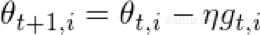其中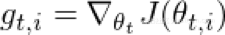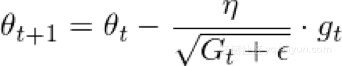RMSprop考虑通过仅使用一定数量的先前梯度来固定递减的学习速率。更新成为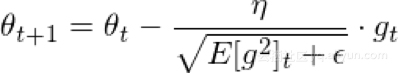其中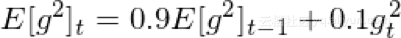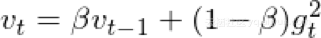v和m可以分别被认为是梯度的第一和第二时刻的估计，因此得到自适应矩估计的名称。当这是第一次使用时，研究人员观察到存在0的固有偏差，他们通过使用以下估计来反驳这一点：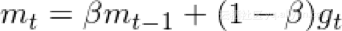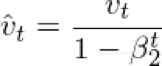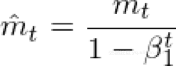1.Â  每个参数和每次迭代的学率都不同；

3.Â  梯度更新使用权重分布矩阵

#### 正则化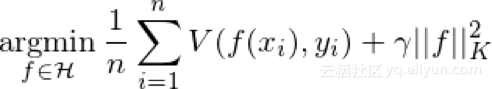#### Dropout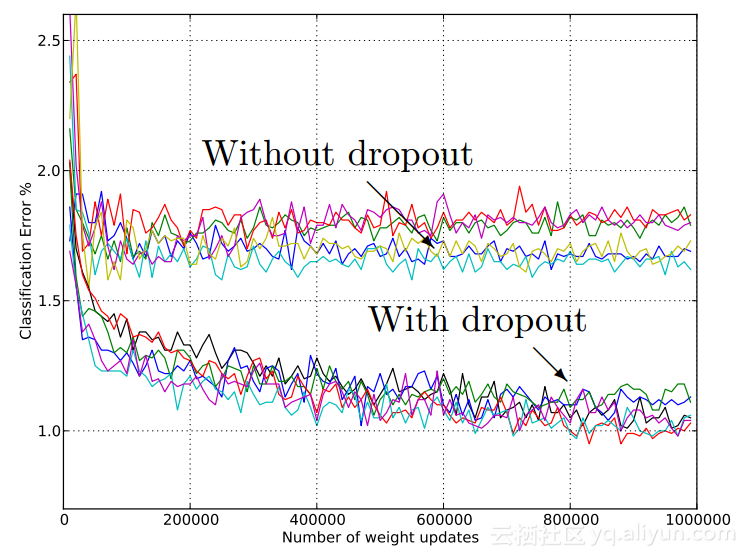#### 5.模型实施

class NeuralNetwork:
def __init__(self, input_shape, stock_or_return):
self.input_shape = input_shape
self.stock_or_return = stock_or_return
def make_train_model(self):
input_data = kl.Input(shape=(1, self.input_shape))
lstm = kl.LSTM(5, input_shape=(1, self.input_shape), return_sequences=True, activity_regularizer=regularizers.l2(0.003),
recurrent_regularizer=regularizers.l2(0), dropout=0.2, recurrent_dropout=0.2)(input_data)
perc = kl.Dense(5, activation="sigmoid", activity_regularizer=regularizers.l2(0.005))(lstm)
lstm2 = kl.LSTM(2, activity_regularizer=regularizers.l2(0.01), recurrent_regularizer=regularizers.l2(0.001),
dropout=0.2, recurrent_dropout=0.2)(perc)
out = kl.Dense(1, activation="sigmoid", activity_regularizer=regularizers.l2(0.001))(lstm2)
model = Model(input_data, out)
# train model
model.fit(train, train_y, epochs=2000)

#### 结果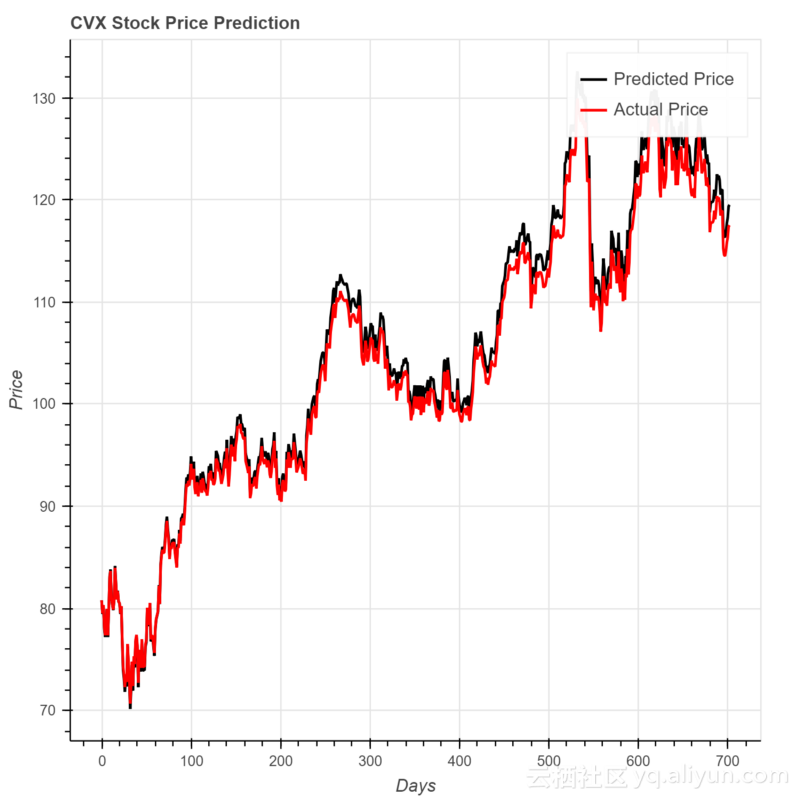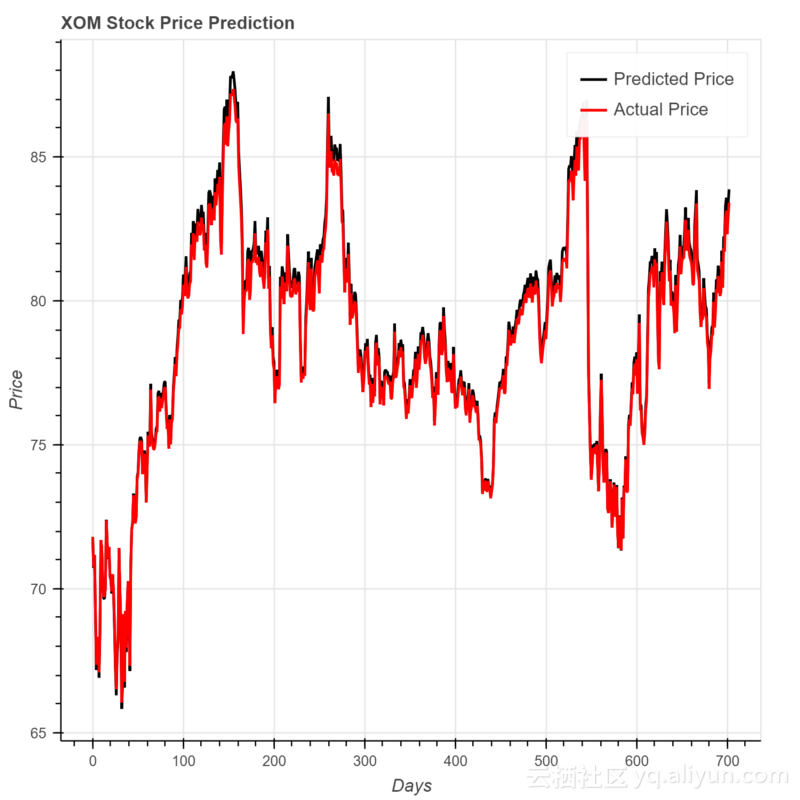#### 结论《Scala机器学习》一一第3章 使用Spark和MLlib
1223 0Html5 学习系列（四）文件操作API

710 01848 0Civil 3D API二次开发学习指南
1831 01224 01799 0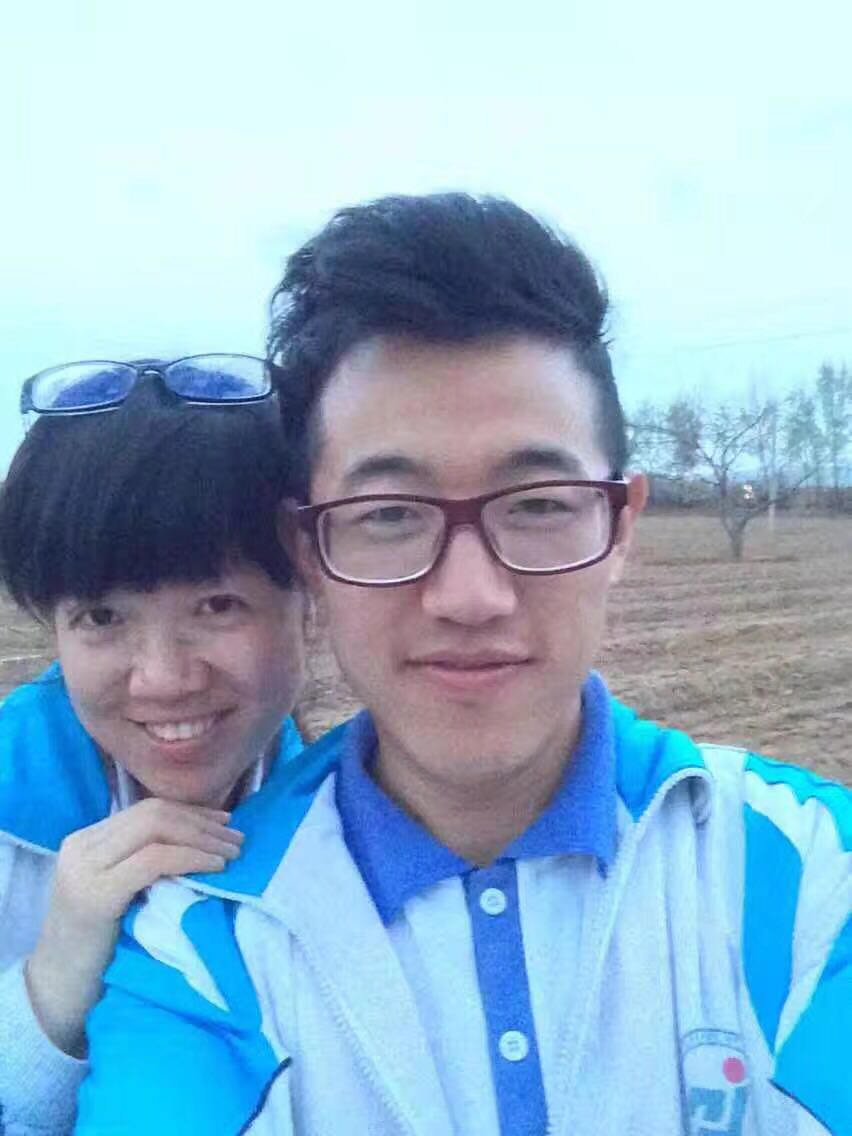453 0ITAA:网络模拟平台+学习资料+学习视频=7.5G(Bit下载)
http://www.gotolab.cn/2008/06/22/itaa-open-vmware/
483 0Asp.Net Web API 2 官网菜鸟学习系列导航[持续更新中]

1415 045 0
【方向】

707

5

JS零基础入门教程（上册）